2022/07/14
697

# Java程式教室(三)：用JAVA寫出擲骰子遊戲## 操作步驟

Step1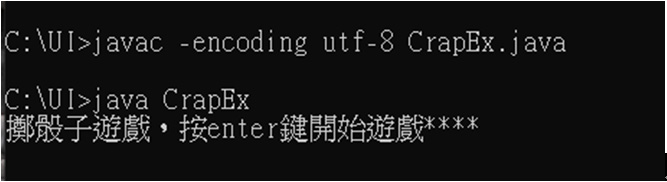Step 2-擲骰子，玩家輸，按任意鍵繼續玩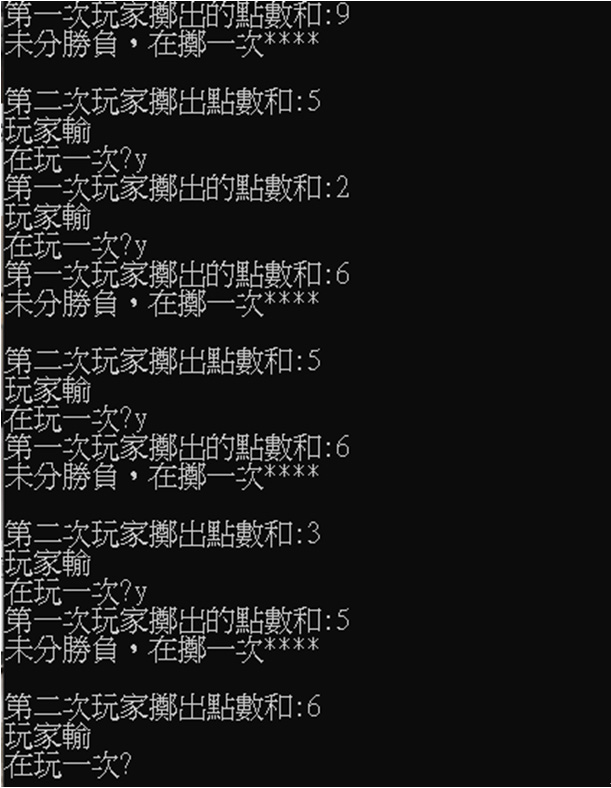Step 3-擲篩子，一直到玩家勝為止，按n結束遊戲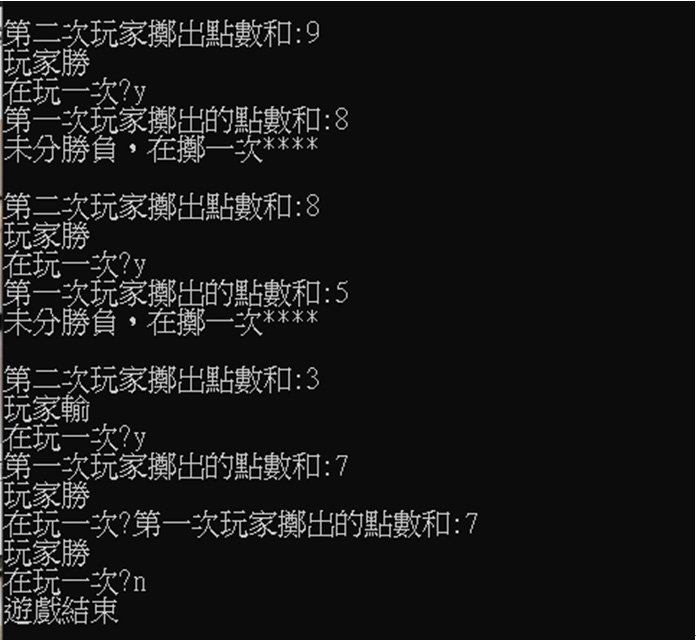## 參考解答:

---

 import java.io.IOException;   class CrapEx {         public static void main(String[] args) throws IOException{                   final int WAN=0,Lost=1,Mid=2;                 boolean flag=true;                 int Outcome=Mid;                 int sum;                 int point=0;                 System.out.println("擲骰子遊戲，按enter鍵開始遊戲****");                 while(true)                 {                         System.in.read();                         if(flag){                                 sum=getPoint();                                 System.out.println("第一次玩家擲出的點數和:"+sum);                                 switch(sum)                                 {                                         case 7:                                         case 11:                                                 Outcome=WAN;                                                 break;                                         case 2:                                         case 3:                                         case 12:                                                 Outcome=Lost;                                                 break;                                         default:                                                 flag=false;                                                 Outcome=Mid;                                                 point=sum;                                                 break;                                      }                           }                         else                         {                                 sum=getPoint();                                   System.out.println("\n第二次玩家擲出點數和:"+sum);                                 if(sum==point)                                 {                                         Outcome=WAN;                                 }                                 else                                 {                                         Outcome=Lost;                                 }                         }                           if(Outcome==Mid)                         {                                   System.out.println("未分勝負，在擲一次****");                         }                         else                         {                                 if(Outcome==WAN)                                 {                                         System.out.println("玩家勝");                                 }                                 else                                 {                                         System.out.println("玩家輸");                                 }                                 System.out.print("在玩一次?");                                   if(System.in.read()=='n')                                 {                                         System.out.println("遊戲結束");                                         break;                                 }                                 flag=true;                         }                 }         }         public static int getPoint()         {                 int roll=((int)(Math.random()*6)+(int)(Math.random()*6));                 if(roll<2)                 {                         roll=2;                 }                   return roll;         }   }

---

>>程式課程試聽索取

>>職訓補助課程江聖榮 老師   專長：   程式設計：從前端(UI front-end)到後端(Back-end)之全端工程師(full-stack)   精通語言：Java、Javascript 前後端框架TOP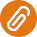你可能有興趣的文章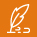熱門標籤